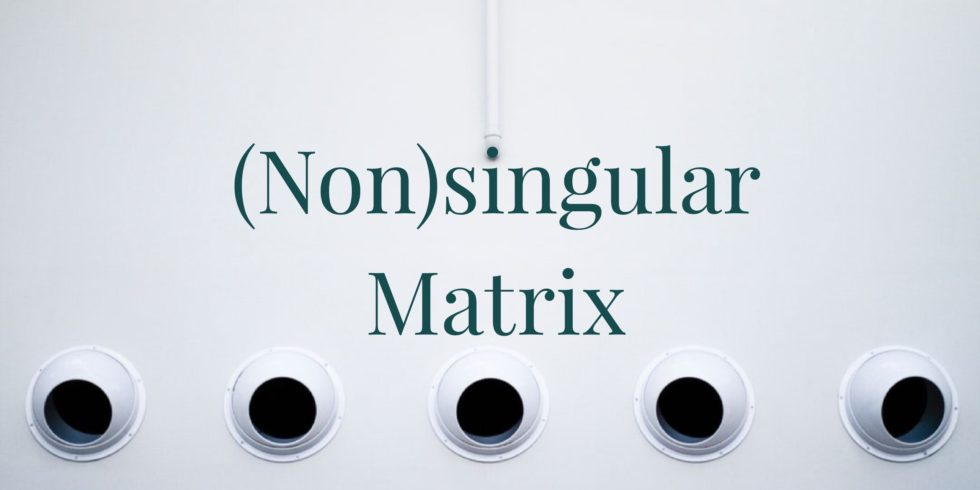# For What Values of $a$, Is the Matrix Nonsingular?## Problem 670

Determine the values of a real number $a$ such that the matrix
$A=\begin{bmatrix} 3 & 0 & a \\ 2 &3 &0 \\ 0 & 18a & a+1 \end{bmatrix}$ is nonsingular.Add to solve later

## Solution.

We apply elementary row operations and obtain:
\begin{align*}
A=\begin{bmatrix}
3 & 0 & a \\
2 &3 &0 \\
0 & 18a & a+1
\end{bmatrix}
\xrightarrow{R_1-R_2}
\begin{bmatrix}
1 & -3 & a \\
2 &3 &0 \\
0 & 18a & a+1
\end{bmatrix}\6pt] \xrightarrow{R_2-2R_1} \begin{bmatrix} 1 & -3 & a \\ 0 &9 &-2a \\ 0 & 18a & a+1 \end{bmatrix} \xrightarrow{R_3-(2a)R_2} \begin{bmatrix} 1 & -3 & a \\ 0 &9 &-2a \\ 0 & 0 & 4a^2+a+1 \end{bmatrix}. \end{align*} From this, we see that the matrix A is nonsingular if and only if the (3, 3)-entry 4a^2+a+1 is not zero. By the quadratic formula, we see that \[a=\frac{-1\pm \sqrt{-15}}{8} are solutions of $4a^2+a+1=0$.

Note that these are not real numbers. Thus, for any real number $a$, we have $4a^2+a+1\neq 0$.

Hence, we can divide the third row by this number, and eventually we can reduce it to the identity matrix.
So the rank of $A$ is $3$, and $A$ is nonsingular for any real number $a$.Add to solve later

### More from my site

#### You may also like...

This site uses Akismet to reduce spam. Learn how your comment data is processed.

###### More in Linear Algebra##### Are Coefficient Matrices of the Systems of Linear Equations Nonsingular?

(a) Suppose that a $3\times 3$ system of linear equations is inconsistent. Is the coefficient matrix of the system nonsingular?...

Close